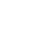# 用Python实战制作一张极具意义的人生进度图

1.享受生活

2.为未来的每个点能够享受生活打下基础

3.创造一些能让自己或他人活得更有趣的东西

## 2.基本使用

K20shores大神的这个库基本包含了我们所有需要使用的元件。

```# 增加事件名称及其时间段
g.add_era('COVID-19', date(2020, 1, 1), date(2020, 7, 1), color="#605041")```

```from lifegraph.lifegraph import Lifegraph, Papersize, Side
from datetime import date

# 以出生日期为起点
birthday = date(1997, 6, 28)
g = Lifegraph(birthday, dpi=300, size=Papersize.A4, max_age=100)

g.show_max_age_label()

# 增加事件名称及其时间段
g.add_era('COVID-19', date(2020, 1, 1), date(2020, 7, 1), color="#605041")

g.save("grid.png")```

```# 标记一个生命事件，可配置文本颜色及显示位置
g.add_life_event('Pythondict\nCreated', date(2019, 7, 31), color="#dc143c", side=Side.LEFT)```

```from lifegraph.lifegraph import Lifegraph, Papersize, Side
from datetime import date

# 以出生日期为起点
birthday = date(1997, 6, 28)
g = Lifegraph(birthday, dpi=300, size=Papersize.A4, max_age=100)

g.show_max_age_label()

# 增加事件名称及其时间段
g.add_era('COVID-19', date(2020, 1, 1), date(2020, 7, 1), color="#605041")
g.add_life_event('Pythondict\nCreated', date(2019, 7, 31), color="#dc143c", side=Side.LEFT)

g.save("grid.png")```

## 3.高级使用

```from lifegraph.lifegraph import Lifegraph, Papersize, Side
from datetime import date

# 以出生日期为起点
birthday = date(1997, 6, 28)
g = Lifegraph(birthday, dpi=300, size=Papersize.A4, max_age=100)

g.show_max_age_label()

# 增加事件名称及其时间段
g.add_era('COVID-19', date(2020, 1, 1), date(2020, 7, 1), color="#605041")
g.add_life_event('Pythondict\nCreated', date(2019, 7, 31), color="#dc143c", side=Side.LEFT)

# 设置背景图

g.save("grid.png")```

```from lifegraph.lifegraph import Lifegraph, Papersize, Side
from datetime import date

# 以出生日期为起点
birthday = date(1997, 6, 28)
g = Lifegraph(birthday, dpi=300, size=Papersize.A4, max_age=100)

g.show_max_age_label()

# 增加事件名称及其时间段
g.add_era('COVID-19', date(2020, 1, 1), date(2020, 7, 1), color="#605041")

# 连接 A B 两个日期
'From A to B',
date(2016, 12, 31), date(2020, 7, 13),
color="#4423fe",
side=Side.LEFT
)

g.save("grid.png")```

​Python实用宝典 (pythondict.com)

# Python 快速绘制画出漂亮的系统架构图

Diagrams  是一个基于Python绘制云系统架构的模块，它能够通过非常简单的描述就能绘制画出可视化架构，其支持使用以下6种云产品的图标：

`AWS``Azure``GCP``Kubernetes``阿里云,` `Oracle Cloud`

```from diagrams import Cluster, Diagram
from diagrams.aws.compute import ECS
from diagrams.aws.database import RDS
from diagrams.aws.network import Route53

with Diagram("Simple Web Service with DB Cluster", show=False):
dns = Route53("dns")
web = ECS("service")

with Cluster("DB Cluster"):
db_master = RDS("master")
db_master - [RDS("slave1"),
RDS("slave2")]

dns >> web >> db_master```

## 1.准备

Windows环境下打开Cmd(开始—运行—CMD)，苹果系统环境下请打开Terminal(command+空格输入Terminal)，准备开始输入命令安装依赖。

`pip install diagrams`

## 2.基本使用与例子

### 2.1 初始化与导出

```from diagrams import Diagram
from diagrams.aws.compute import EC2

with Diagram("Simple Diagram"):
EC2("web")```

`with Diagram("Simple Diagram", outformat="jpg", filename="my_diagram")`

### 2.2 节点类型

```from diagrams import Diagram
from diagrams.aws.compute import EC2
from diagrams.aws.database import RDS
from diagrams.aws.network import ELB
from diagrams.aws.storage import S3

# show参数表示是否自动打开图像
with Diagram("Web Services", show=False):
ELB("lb") >> EC2("web") >> RDS("userdb") >> S3("store")
ELB("lb") >> EC2("web") >> RDS("userdb") << EC2("stat")
(ELB("lb") >> EC2("web")) - EC2("web") >> RDS("userdb")```

>> 表示从左到右的数据流
<< 表示从右到左的数据流
– 表示没有箭头的数据流

```from diagrams import Diagram
from diagrams.aws.compute import EC2
from diagrams.aws.database import RDS
from diagrams.aws.network import ELB

with Diagram("Workers", show=False, direction="TB"):
lb = ELB("lb")
db = RDS("events")
lb >> EC2("worker1") >> db
lb >> EC2("worker2") >> db
lb >> EC2("worker3") >> db
lb >> EC2("worker4") >> db
lb >> EC2("worker5") >> db```

```from diagrams import Diagram
from diagrams.aws.compute import EC2
from diagrams.aws.database import RDS
from diagrams.aws.network import ELB

with Diagram("Grouped Workers", show=False, direction="TB"):
ELB("lb") >> [EC2("worker1"),
EC2("worker2"),
EC2("worker3"),
EC2("worker4"),
EC2("worker5")] >> RDS("events")```

### 2.3 集群块

```from diagrams import Cluster, Diagram
from diagrams.aws.compute import ECS
from diagrams.aws.database import RDS
from diagrams.aws.network import Route53

with Diagram("Simple Web Service with DB Cluster", show=False):
dns = Route53("dns")
web = ECS("service")

with Cluster("DB Cluster"):
db_master = RDS("master")
db_master - [RDS("slave1"),
RDS("slave2")]

dns >> web >> db_master```

```from diagrams import Cluster, Diagram
from diagrams.aws.compute import ECS, EKS, Lambda
from diagrams.aws.database import Redshift
from diagrams.aws.integration import SQS
from diagrams.aws.storage import S3

with Diagram("Event Processing", show=False):
source = EKS("k8s source")

with Cluster("Event Flows"):
with Cluster("Event Workers"):
workers = [ECS("worker1"),
ECS("worker2"),
ECS("worker3")]

queue = SQS("event queue")

with Cluster("Processing"):
handlers = [Lambda("proc1"),
Lambda("proc2"),
Lambda("proc3")]

store = S3("events store")
dw = Redshift("analytics")

source >> workers >> queue >> handlers
handlers >> store
handlers >> dw```

### 2.4 自定义线的颜色与属性

```Edge(color="firebrick", style="dashed", label="test")
# 火砖色的虚线，备注为test```

```from diagrams import Cluster, Diagram, Edge
from diagrams.onprem.analytics import Spark
from diagrams.onprem.compute import Server
from diagrams.onprem.database import PostgreSQL
from diagrams.onprem.inmemory import Redis
from diagrams.onprem.logging import Fluentd
from diagrams.onprem.monitoring import Grafana, Prometheus
from diagrams.onprem.network import Nginx
from diagrams.onprem.queue import Kafka

with Diagram(name="Advanced Web Service with On-Premise (colored)", show=False):
ingress = Nginx("ingress")

metrics = Prometheus("metric")
metrics << Edge(color="firebrick", style="dashed") << Grafana("monitoring")

with Cluster("Service Cluster"):
grpcsvc = [
Server("grpc1"),
Server("grpc2"),
Server("grpc3")]

with Cluster("Sessions HA"):
master = Redis("session")
master - Edge(color="brown", style="dashed") - Redis("replica") << Edge(label="collect") << metrics
grpcsvc >> Edge(color="brown") >> master

with Cluster("Database HA"):
master = PostgreSQL("users")
master - Edge(color="brown", style="dotted") - PostgreSQL("slave") << Edge(label="collect") << metrics
grpcsvc >> Edge(color="black") >> master

aggregator = Fluentd("logging")
aggregator >> Edge(label="parse") >> Kafka("stream") >> Edge(color="black", style="bold") >> Spark("analytics")

ingress >> Edge(color="darkgreen") << grpcsvc >> Edge(color="darkorange") >> aggregator```

## 3.总结

¥1¥5¥10¥20¥50¥100¥200 自定义

​Python实用宝典 ( pythondict.com )

# Blender, 用Python绘制宇宙飞船的3D建模软件

Blender是一个免费的开源3D计算机图形软件工具集，用于创建动画电影视觉效果，艺术品，3D打印模型，动态图形，交互式3D应用程序和计算机游戏

## 快来生成你的宇宙飞船

1. 安装Blender 2.76以上：

2. 下载作者的生成代码（也就是已经和Blender做好API联调的代码）。

3.1 点击菜单栏里的 Edit — 选择Preferences.

3.3 载入后记得勾选插件。

4. 使用快捷键shift+F5进入3D视图

5. 使用快捷键shift+A，这时候鼠标右键会弹出选项，在mesh中选择 Spaceship即可生成你的宇宙飞船！

​Python实用宝典 (pythondict.com)

# 7行代码 Python热力图可视化分析缺失数据处理

## 1.安装所需要的第三方Python库

```pip install seaborn
pip install pandas
pip install matplotlib```

## 2.Python加载数据

```import pandas as pd
import numpy as np
import seaborn
import matplotlib

## 3.Python构造热力图

```seaborn.heatmap(data.isnull(), yticklabels=False, cbar=False, cmap='viridis')
# 热力图，当data中有空值时标为黄色
matplotlib.pyplot.tight_layout()
matplotlib.pyplot.show()```

seaborn.heatmap用于生成热力图，其会检查data中的每个单元格，如果为空则标记为黄色，cmap为颜色图谱，viridis即蓝-绿-黄. 此外，matplotlib.pyplot主要用于负责展示图片。如果你需要知道seaborn更详细的参数文档，可以阅读这篇文章

```import pandas as pd
import seaborn
import matplotlib
seaborn.heatmap(data.isnull(), yticklabels=False, cbar=False, cmap='viridis')
matplotlib.pyplot.tight_layout()
matplotlib.pyplot.show()```

​Python实用宝典 (pythondict.com)

# 精美的数据分析图!教你使用Python的Plotly库

plotly 是目前已知的Python最强绘图，它比上次我们讲的echarts还强大许多许多，它的绘制通过生成一个web页面得到，而且支持调整图像大小后保存，而且还能支持可动态调节的页面，方便python web端的开发。

### 一、安装

Windows环境下打开Cmd(开始—运行—CMD)，苹果系统环境下请打开Terminal(command+空格输入Terminal)，准备开始输入命令安装依赖。

`pip install plotly`

### 二、使用例子

data_frame：数据，需要以DataFrame格式。

color: 可指定每一列的特定颜色。

color_continuous_scale: 构建连续的颜色

https://plotly.github.io/plotly.py-docs/generated/plotly.express.parallel_categories.html

```# 文件命名为：test.py
import plotly.express as px
tips = px.data.tips()
fig = px.parallel_categories(tips, color="size", color_continuous_scale=px.colors.sequential.Inferno)
fig.show()```

```import plotly.express as px
gapminder = px.data.gapminder()
fig = px.scatter(gapminder.query("year==2007"), x="gdpPercap", y="lifeExp", size="pop", color="continent",
hover_name="country", log_x=True, size_max=60)
fig.show()```

### 三、保存

```import plotly.offline as offline
offline.plot(figure_or_data = fig, image = 'png', image_filename='plot_image', output_type='file', image_width=800, image_height=600, validate=False) ```

```# 文件命名为：test.py
import plotly.express as px
import plotly.offline as offline
tips = px.data.tips()
fig = px.parallel_categories(tips, color="size", color_continuous_scale=px.colors.sequential.Inferno)
fig.show()

offline.plot(figure_or_data = fig, image = 'png', image_filename='plot_image',
output_type='file', image_width=800, image_height=600, validate=False)```

### 四、其他功能

1. 共享：如果你希望能和其他人共享图片，而自己又不会做web开发，没关系，plotly也提供了解决方案，这个时候我们需要安装一个新的包叫chart_studio，然后使用官方给定的API进行在线绘制，就能保存到你的plotly个人网页中了！ 详细可见官方教程
2. 添加背景图：你还可以给你的图片添加背景图！而且操作非常简单。
3. 添加你的LOGO：添加你个人独特的LOGO也是支持的！

https://plot.ly/python/images/ 你可以找到这2和3的相应教程！

​Python实用宝典 (pythondict.com)

# python 画雪花 —科赫曲线的实现

```import turtle
def koch(t,n):
#定义一个函数 科赫曲线，完成绘画功能
if n < 5 :
t.fd(n)
return
m = n/3
koch(t,m)
t.lt(60)
koch(t,m)
t.rt(120)
koch(t,m)
t.lt(60)
koch(t,m)

def snowflake(t, n):
# 画一朵雪花，每一边都是一个科赫曲线
for i in range(3):
koch(t,n)
t.rt(120)

bob = turtle.Turtle()
bob.color('black')
bob.penup() # 画笔提起（不能画）
bob.goto(-150,90) #去到这个点
bob.pendown() # 画笔落下（开始画）
snowflake(bob,300) # 调用函数开始画雪花
turtle.mainloop() ```

1. 将线段分成三等份（AC,CD,DB）
2. 以CD为底，向外（内外随意）画一个等边三角形DMC
3. 将线段CD移去
4. 分别对AC,CM,MD,DB重复1~3。